# AlphaZero

Posted by lili on

## 简介

AlphaZero和前面的AlphaGo Zero基本一样，只不过把它用于了象棋等其它棋类游戏（当然也可以用于围棋）。下面我们来看它和AlphaGo Zero的区别。

1. 对于围棋，结果只有胜负，但是象棋有平局(z=0)
2. 对于围棋，AlphaGo Zero利用了对称性，而AlphaZero不使用它来增强数据
3. AlphaGo Zero得到新的模型之后会和当前最优的比赛，胜率超过55%才替换当前模型，而AlphaZero没有这个步骤，直接更新模型，用新的模型来自对弈
4. AlphaGo Zero的超参数使用贝叶斯优化来选择，而AlphaZero重用AlphaGo Zero的超参数，对于象棋也使用围棋的超参数

## 图解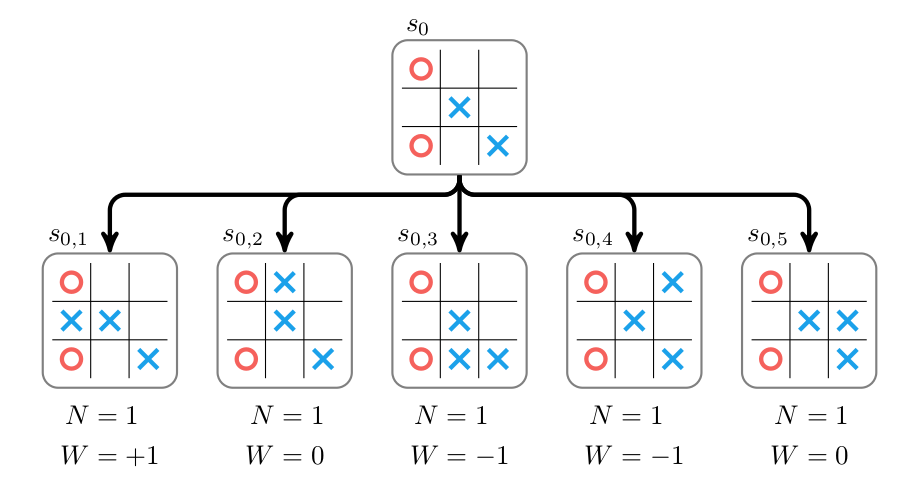图：Rollout其它叶子节点

$U_i = \frac{W_i}{N_i} + c\sqrt{\frac{\ln N_p}{N_i}}$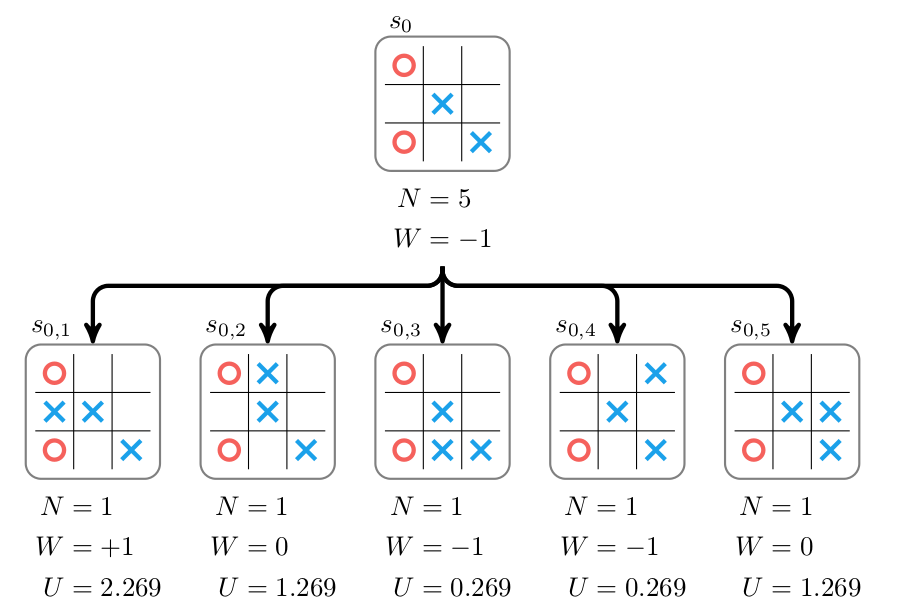图：第二次MCTS的Selection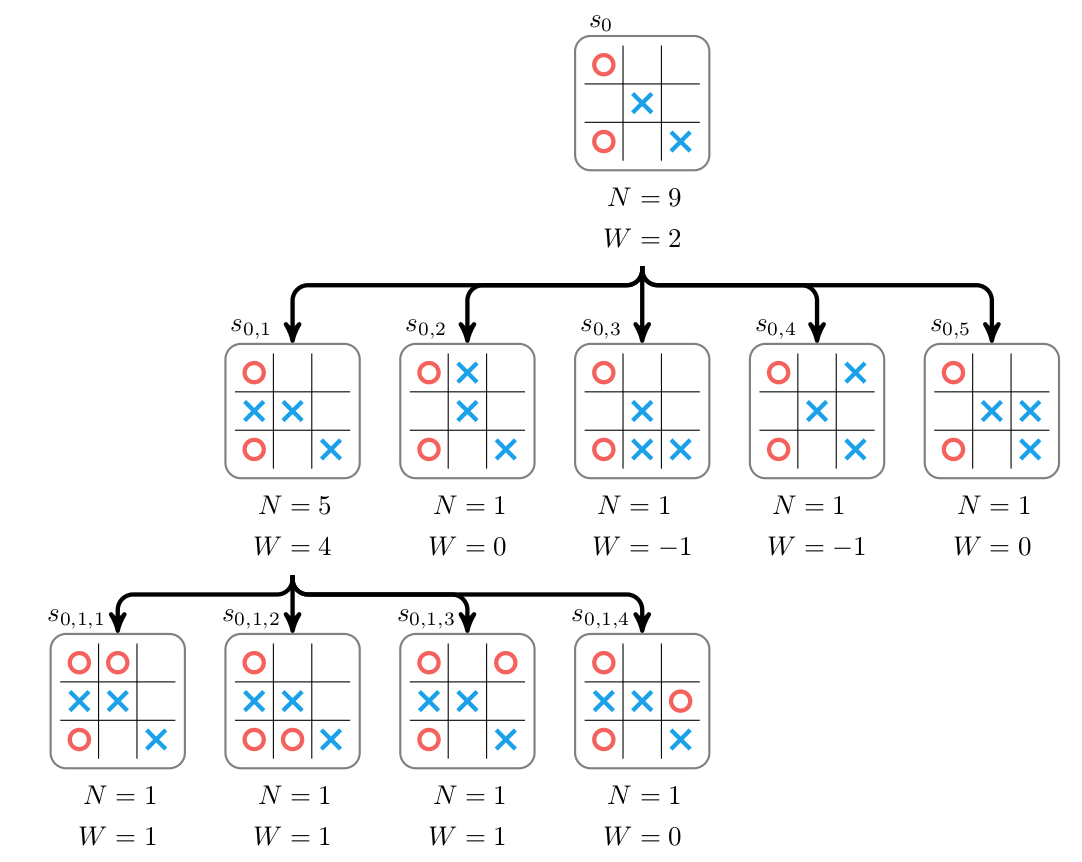图：第二次MCTS的Expand和Evaluation

$U_i = \frac{W_i}{N_i} + c P_i\sqrt{\frac{\ln N_p}{1 + N_i}}$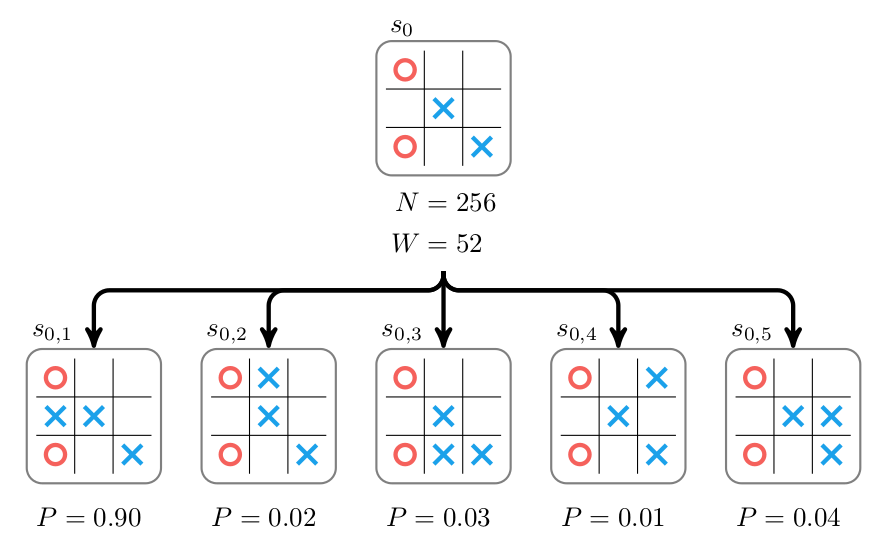图：加入Policy Network去掉rollout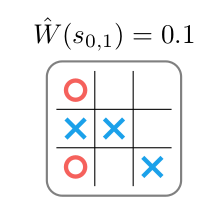图：用Value Network去rollout图：AlphaZero的Expand和Evaluation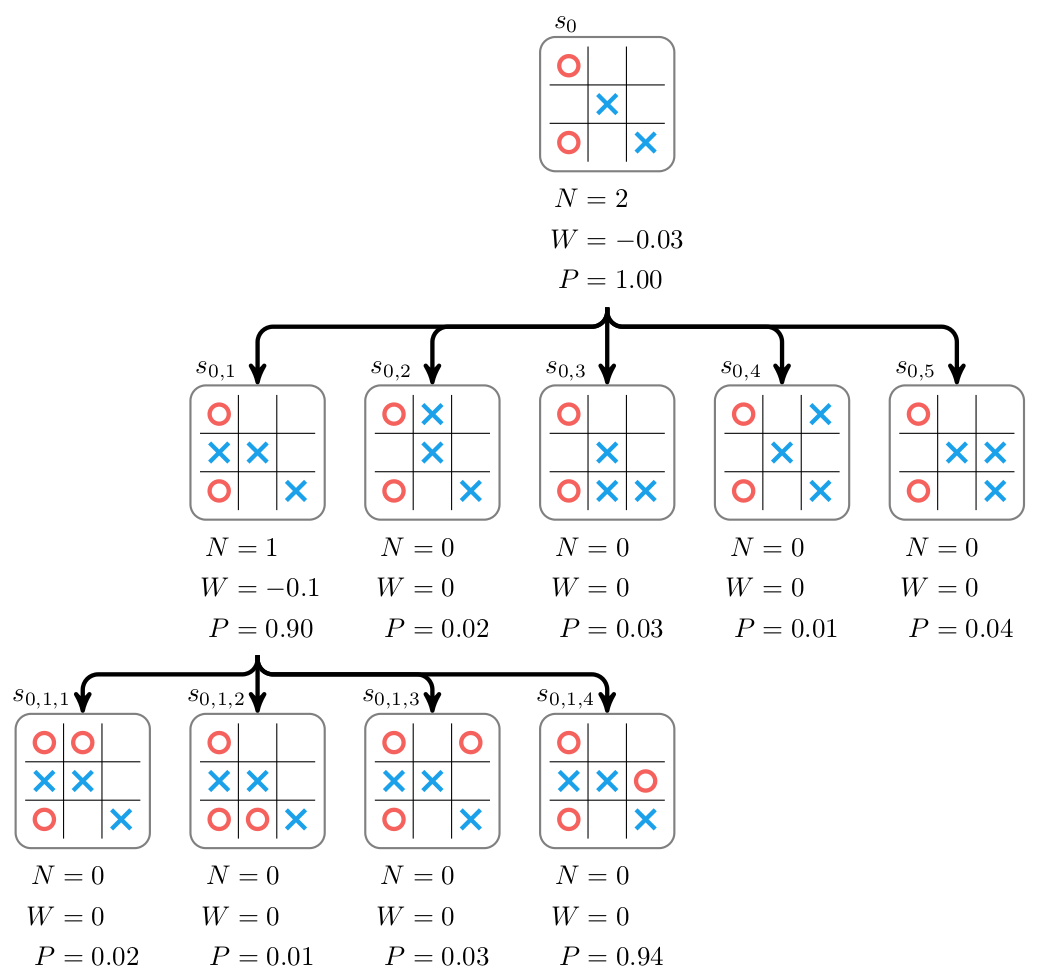图：AlphaZero的Expand和Evaluation

$\pi_i=N(s,a_i)^{1/\tau}$

$(W - Z)^2 + {\boldsymbol{\mathbf{\pi}}}^\top \ln\boldsymbol{\mathbf{p}} + \lambda \|\boldsymbol{\mathbf{\theta}}\|_2^2$

## 实验结果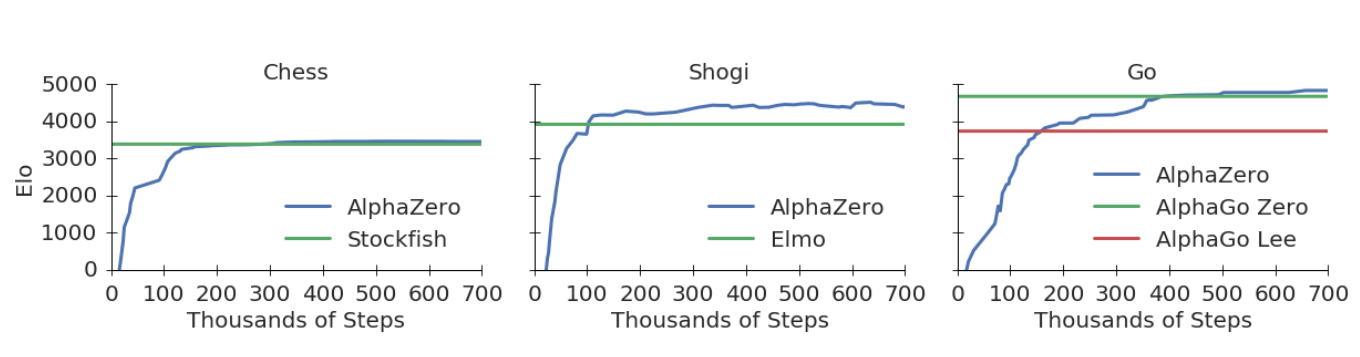图：棋力随训练的迭代次数的变化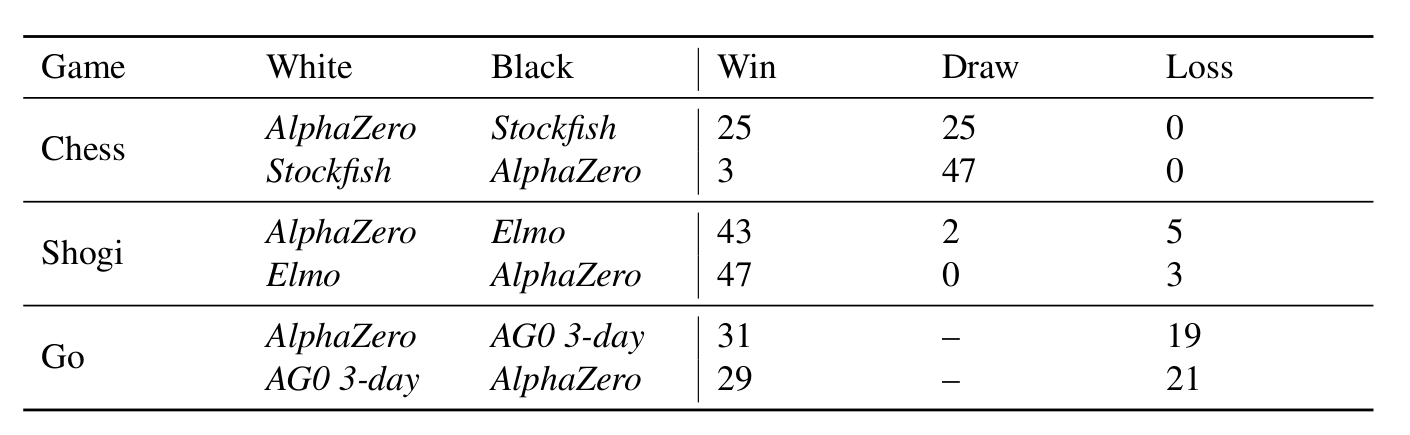图：AlphaZero和顶尖软件的比赛

## 实现细节

### 状态表示

AlphaZero的状态表示如下图所示。其中围棋和前面的AlphaGo Zero完全一样。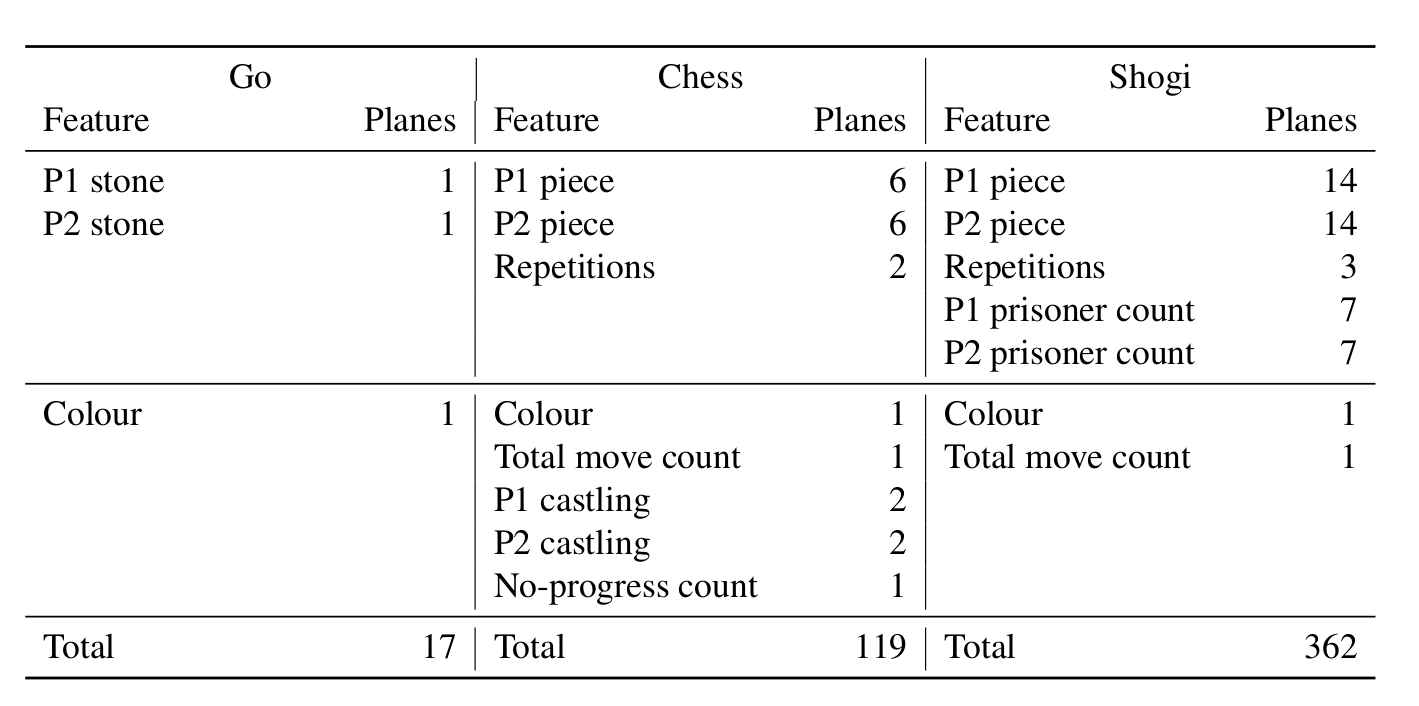图：AlphaZero的状态表示